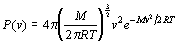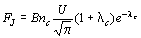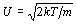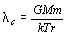Jean’s Escape

When a gas is at a certain temperature not all of the particles in that gas are moving at the same velocity. While the majority of the particles are moving at or near the speed that corresponds to the temperature of the gas, some will hardly be moving at all and some small fraction of them will be moving at very high speeds. The exact distribution of probabilities for different velocities is called a Maxwellain after James Clerk Maxwell who published the form in 1852. The probability distribution is of the form, where v is the molecular speed, T is the temperature, M is the molar mass of the gas, and R is the gas constant. Figure 1 shows a graph of this distribution. Notice how there is a long extended tail to it indicating that a small portion of the particles will have a very high velocity.

If a particle gets up to a speed greater than the escape velocity of the planet then it can escape assuming that it doesn’t run into any other particles that might slow it down. This second condition is satisfied in the exosphere of the atmosphere. When a particle in the exosphere reaches a speed greater than the escape velocity in the upward direction it will escape the planets gravitational attraction and leave the atmosphere.

To show how this happens here are a few simple simulations of particles in a box. The boxes in these simulations have floors that are kept at a constant temperature, walls that are insulated, and a ceiling that is sticky. When a particle becomes stuck to the ceiling it is considered to have escaped. Just click the start button to begin any of the simulations. Unless you are on a very fast computer running a very efficient JVM it is also recommended that you stop the simulations when you are done viewing one and want to move onto the next.

The first simulation shows particles of two different sizes with no collisions. Though all the particles begin the simulation at about the same height, it doesn’t take long for the smaller particles to gain energy from the floor and begin bouncing to much higher levels. The mass rations of the particles is 1:4 (comparable to helium and atom oxygen) so the small particles should have a maximum velocity that is twice that of the larger ones and bounce four times as high. However, no matter how long you wait, none of the particles should ever reach the ceiling. This is because without collisions the Maxwellian distribution of velocities doesn’t form. Instead all of the particles remain at the velocity that corresponds to the temperature of the floor.

Model 1: A simulation of non-colliding particles of two different sizes. Press the start button to watch the simulation. The bottom boundary is kept at a constant temperature and the walls are insulating. Note that the smaller particles come closer to escaping than the larger ones, but without collisions they can't quite reach the top of the box.

The second simulation simply changes all of the larger particles into smaller ones, but adds collisions. In this case a distribution of velocities is produced and some particles can escape from the system. Because the number of particles is so small (20), one does not expect to get a truly Maxwellian distribution at any given time. However, by summing over many time steps a nice Maxwellian form should appear. This form will be somewhat cut short on the tail though because particles that reach the highest velocities tend to leave the system. In this simulation it is possible to have all but one of the particles escape. If the floor were not held at a constant temperature, this would not be the case because every particle that escapes removes energy from the system. If the floor were not adding that energy back into the system, at some point the remaining particles would have a total energy lower than the escape energy for one particle, and therefore no more would be able to escape. The constant temperature floor, however, is more like the real system, however, because the Sun does indirectly provide the energy to keep the system at about the same temperature so that such energy loss in not seen.

Model 2: In the model the large particles have been converted to smaller ones and collisions are being processed. Note that with the collisions these particles can reach the top of the box, effectively escaping the system.

The third simulation returns to having both large and small particles but with collisions. Again a distribution of particle velocities is created. One can easily see that the larger particles tend to have lower speeds than their smaller counterparts and the smaller particles are ejected much more quickly. Actually, I have never seen one of the larger particles escape, but from the relation given in the next paragraph it should only take about 55 times as long for the larger particles to escape as it does for the smaller ones. However, because the escape time for a small particles seems to be on the order of 30 seconds it is quite possible that one would have to let this simulation run for nearly a half hour to see just one of the large particles escape.

Model 3: Here the large particles have been reintroduced with collisions on. The small particles still readily escape, but even with collisions the escape of the larger particles is very rare.

An analytic form for the rate of escape can be given by the expression, where B is a constant of order 0.5, nc is the number density at the exobase,is the most probable speed of the Maxwellian, andis the escape parameter at the exobase. For the case of hydrogen in the Earth’s exosphere normal values are T=900 K, l c=8, nc=105 cm-3, B=0.75 m and FJ=6*107 cm-2 s-1. It is important to notice where the mass of the particles comes into this equation and that is in l c. It then becomes immediately apparent that the flux rate decays exponentially with the mass of the particle being considered. For this reason Jean’s escape is not a significant factor on the Earth for any species much heavier than Helium.

In the atmospheric model Jean’s escape was implemented by approximating the height of the exobase and applying this formula to the values of the parameters at that elevation. The calculated flux rate was then removed from all of the bins at or above the exobase. This equation takes into account the time it takes for more particles to "move" into the tail region of the distribution. However, in many real systems this rate is actually limited by how quickly the supply of particles in the exosphere can be replenished. That is to say that it is limited by spatial diffusion instead of velocity diffusion. To account for this in the model, the time step is adjusted so that the loss process can’t remove more atmosphere than is there.